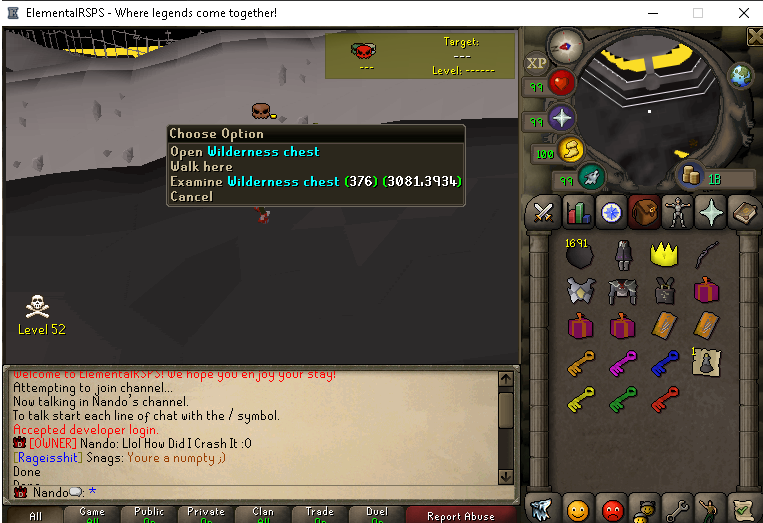What's new
Elemental RSPS - Best Runescape Private Server 2022

Register a free account today to become a member! Once signed in, you'll be able to participate on this site by adding your own topics and posts, as well as connect with other members through your own private inbox!

Status
Not open for further replies.

####Nandox

##### Owner
Staff member
Wilderness keys are an addition to wildy slayer, Since alot of people don't know what they are i will make this short topic about it.
There are 6 different wilderness keys i will briefly explain them:Every key can be used north of the mage arena:The droprates of the wilderness keys are calculated like this:
Code:
``````int chance = ((hp / 10));
if (chance >= 40)
chance = 40;
if (RandomUtility.getRandom(200) < chance) {``````
Basicly what this does is divide the npc hp by 100, if chance gets higher then 40 max chance is 40.
A Random number generator rolls between 0-200 and if it falls on 40 or lower you'll recieve an Green Key.

Green Key:
Code:
``````int chance = ((hp / 10));
if (chance >= 40)
chance = 40;
if (RandomUtility.getRandom(200) < chance) {``````
Yellow Key:
Code:
``````int chance = ((hp / 30));
if (chance >= 40)
chance = 40;
if (RandomUtility.getRandom(200) < chance) {``````
Orange Key
Code:
``````int chance = ((hp / :70);
if (chance >= 30)
chance = 30;
if (RandomUtility.getRandom(200) < chance) {``````
Red Key:
Code:
``````        int chance = ((hp / 110));
if (chance >= 20)
if (RandomUtility.getRandom(100) < chance) {;``````
Purple key:
Code:
``````        int chance = ((hp / 200));
if (chance >= 30)
chance = 30
if (RandomUtility.getRandom(200) < chance) {;``````
Blue key:
Code:
``````        int chance = ((hp / 300));
if (chance >= 15)
chance = 15
if (RandomUtility.getRandom(100) < chance) {;``````

Last edited:
Status
Not open for further replies.